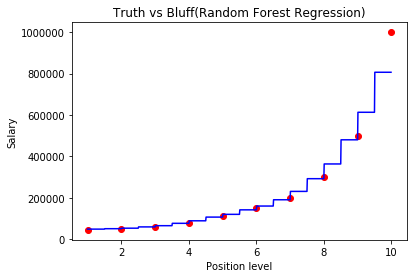×

Search anything:

# Random Forests using Scikit-learn

#### Machine Learning (ML)Get this book -> Problems on Array: For Interviews and Competitive Programming

Reading time: 30 minutes | Coding time: 10 minutes

In this article, we will implement random forest in Python using Scikit-learn (sklearn). Random forest is an ensemble learning algorithm which means it uses many algorithms together or the same algorithm multiple times to get a more accurate prediction.

Random forest intuition

1. First of all we will pick randomm data points from the training set.
2. Build a decision tree associated to the selected m data points.
3. Choose the number of decision trees you want to build and repeat steps 1 and 2.
4. For a new data point say k, make each one of the decision trees predict the value of y for k and assign k, the average of all the predicted y values.

In random forest regression, there are many decision trees making prediction for the dependent variable.

Now let's build a Random forest regression model.

``````#importing the libraries
import numpy as np
import pandas as pd
import matplotlib.pyplot as plt
%matplotlib inline
``````

Importing the libraries numpy for linear algebra matrices, pandas for dataframe manipulation and matplotlib for plotting and we have written %matplotlib inline to view the plots in the jupyter notebook itself.

``````#importing the dataset
``````

We are using the same dataset, in which we want to predict the salary for a new employee whose level of experience is 6.5 and he said that the previous company paid him 160000 and he wants a higher salary and we have got some data which has three columns- Position, Level and Salary. then here we will use random forest regression to predict his salary based on the data we have.

``````dataset.info
``````

This is the dataset.

``````    Position            Level  Salary
1  Junior Consultant      2    50000
2  Senior Consultant      3    60000
3            Manager      4    80000
4    Country Manager      5   110000
5     Region Manager      6   150000
6            Partner      7   200000
7     Senior Partner      8   300000
8            C-level      9   500000
9                CEO     10   1000000
``````

Now we divide our dataset into X and y, where X is the independent variable and y is the dependent variable.

``````X=dataset.iloc[:,1:2].values
y=dataset.iloc[:,2].values
``````
``````#fitting the random forest regression to the dataset
from sklearn.ensemble import RandomForestRegressor
regressor=RandomForestRegressor(n_estimators=300,random_state=0)
regressor.fit(X,y)
``````

We are training the entire dataset here and we will test it on any random value. Suppose the new employee said he has a experience of 6.5 years so we will predict his salary based on that.

``````#predicting the results
from numpy import array
y_pred=regressor.predict(array([[6.5]]))
``````

Now let's check what is the predicted salary for the new employee.

``````y_pred
``````

It returns 160333.33333333,Which is quite accurate and almost equal to the real value.

Now let's visualize the results

``````#visualising the Regression results
X_grid=np.arange(min(X),max(X),0.01)
X_grid=X_grid.reshape(len(X_grid),1)
plt.scatter(X,y,color='red')
plt.plot(X_grid,regressor.predict(X_grid),color='blue')
plt.title('Truth vs Bluff(Random Forest Regression)')
plt.xlabel('Position level')
plt.ylabel('Salary')
plt.show()
``````So, our prediction with random forest is quite accurate than decision trees. Random forest predicts quite better than decision trees in this case.